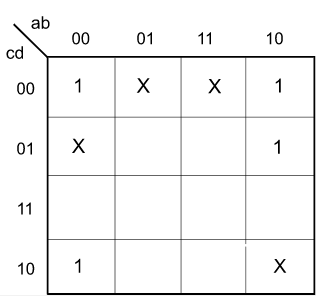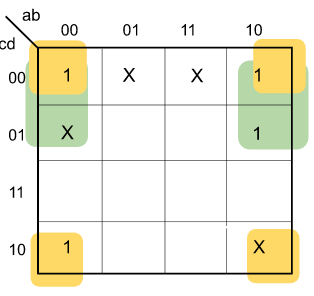# Digital Logic & Number representation

 Question 1
In the following truth table, V = 1 if and only if the input is valid.What function does the truth table represent?Priority encoderDecoderMultiplexerDemultiplexer

Question 1-Explanation:
Since there are more than one outputs and number of outputs is less than inputs, it is a Priority encoder V=1 when input is valid and for priority encoder it checks first high bit encountered. Except all are having at least one bit high and ‘x’ represents the “don’t care” as we have found a high bit already. So answer is (A).
 Question 2
Which one of the following expressions does NOT represent exclusive NOR of x and y?xy+x'y'x⊕y'x'⊕yx'⊕y'

Question 2-Explanation:
By Definition of XNOR,So Option-A is correct. Also by Definition of XOR,Option-B isSo Option-B is also correct. Option-C isOption-C is also correct. Option-D x'⊕y' = x''y' + x'y'' = xy' + x'y = x⊕y ≠ x⊙y Therefore option (D) is false. This explanation is provided by Chirag Manwani.
 Question 3XX+YX xor YY

Question 3-Explanation:
The value of f(X, Y) is same as X for all input pairs. Also sum of product form of expression we get,
= XY’+XY
= X(Y’+Y)
= X *1
= X 
We see from truth table –
Column x = f(x,y)
So ,
f(x,y)=x

Option (A) is correct.
 Question 4

What is the minimal form of the Karnaugh map shown below? Assume that X denotes a don’t care term.b'd'b'd' + b'c'b'd' + a'b'c'd'b'd' + b'c' + c'd'

Question 4-Explanation:

There are two prime implicants in the following K-Map-Prime Implicant highlighted in Green =Prime Implicant highlighted in Orange =So the Boolean expression is-Therefore option (B) is correct.

 Question 5

What is the minimal form of the Karnaugh map shown below? Assume that X denotes a don’t care term.b'd'b'd' + b'c'b'd' + a'b'c'd'b'd' + b'c' + c'd'

Question 5-Explanation:

There are two prime implicants in the following K-Map-Prime Implicant highlighted in Green =Prime Implicant highlighted in Orange =So the Boolean expression is-Therefore option (B) is correct.

 Question 6
Which one of the following circuits is NOT equivalent to a 2-input XNOR (exclusive NOR) gate?ABCD

Question 6-Explanation:
All options except D produce XOR as described below :Question 7
The simplified SOP (Sum Of Product) form of the boolean expression (P + Q' + R') . (P + Q' + R) . (P + Q + R') is(P'.Q + R')(P + Q'.R')(P'.Q + R)(P.Q + R)

Question 7-Explanation:
See following : (P+Q'+R').(P+Q'+R).(P+Q+R') =From the K-map, POS form is : P + Q'.R'
 Question 8

Consider the following circuit involving three D-type flip-flops used in a certain type of counter configuration.If at some instance prior to the occurrence of the clock edge, P, Q and R have a value 0, 1 and 0 respectively, what shall be the value of PQR after the clock edge?000001010011

Question 8-Explanation:

P' = R Q' = (P + R)' R' = QR' Given that (P, Q, R) = (0, 1, 0), next state P', Q', R' = 0, 1, 1 ----------------------------------------------------------------------------------------------- D flip flop truth table

Initially (p,q,r) =(0,1,0) D for p=R D for q=NOT(p xor r) D for r= (not)r.q So Q(t+1) for(p,q,r) p=>r=0 so p=0 q=> NOT(p xor r) => 1      so q=1 r=>(not)r.q => 1         so r=1 (p, q, r) = (0, 1, 1) Alternative approach - Truth table of a D Flip-Flop-By looking at the circuit diagram, it is clear that the boolean expressions of P, Q, and R are- Here the subscript t refers to the current clock cycle, and the subscript (t+1) refers to the next clock cycle.[Tex]Q_{Q(t+1)} = Q_{t+1} = R_{t}' P_{t}'  [/Tex][Tex]\begin{tabular}{|c|c|c|} \hline D_{t} & Q_{t+1}\\ \hline 0 & 0\\ \hline 1 & 1\\ \hline \end{tabular}  [/Tex]This explanation is provided by Chirag Manwani

 Question 9

Consider the data given in previous question. If all the flip-flops were reset to 0 at power on, what is the total number of distinct outputs (states) represented by PQR generated by the counter?3456

Question 9-Explanation:

There are four distinct states, 000 → 010 → 011 → 100 (→ 000) so the answer is B

 Question 10
The minterm expansion of f(P, Q, R) = PQ + QR' + PR' ism2 + m4 + m6 + m7m0 + m1 + m3 + m5m0 + m1 + m6 + m7m2 + m3 + m4 + m5

Question 10-Explanation:
K-map,= PQ + QR’ + PR’
= PQ(R+R’) + (P+P’)QR’ + P(Q+Q’)R’
= PQR + PQR’ +PQR’ +P’QR’ + PQR’ + PQ’R’
= PQR(m7) + PQR'(m6)+P’QR'(m2) +PQ’R'(m4)
= m2 + m4 + m6 + m7 
Option (A) is correct.
There are 267 questions to complete.
• Last Updated : 27 Sep, 2021